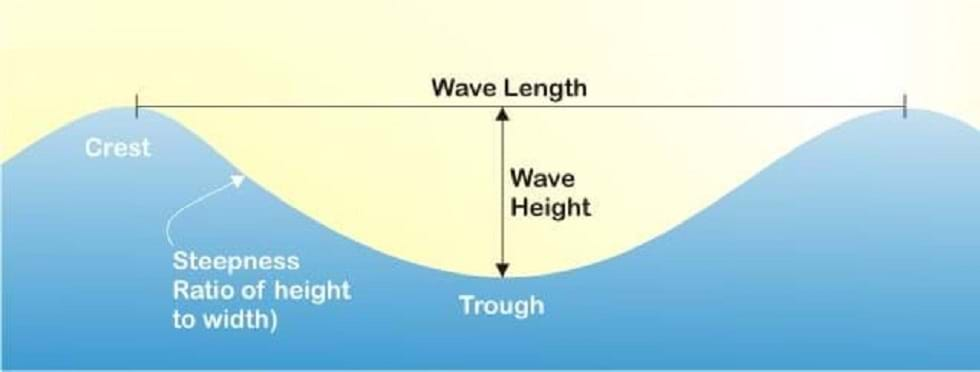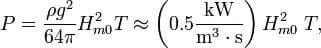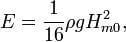# Wave Energy Calculator

#### How to Calculate Wave Energy## Wave Power Watt Per Meter

### Power of a Wave FormulaP = ρ*g2*T*H2/(64*π), [W/m]
H - the vertical distance between crest and trough of the wave, meters;
T - the period of wave, seconds;
g - the acceleration of gravity, m/s2;
ρ - the mass density of sea water, kg/m3;
π - 3.14159

The power from this formula represents the wave energy flux the transport rate of wave energy.

So with this formula, you can now determine the power that can get extracted per meter of crest of the wave.

While the larger a wave is the more power, it will generally have. But wave power also gets determined by the speed of the wave, the waters density, and its wavelength.

## Wave Energy Watt Per Square Meter

### Energy of a Wave FormulaE = ρ*g*H2/16, [J/m2]
g - the acceleration of gravity, m/s2;
ρ - the mass density of sea water, kg/m3;
H - the vertical distance between crest and trough of the wave, meters;